# Hypergeometrical Universe

## Electron-Proton Fundamental Dilator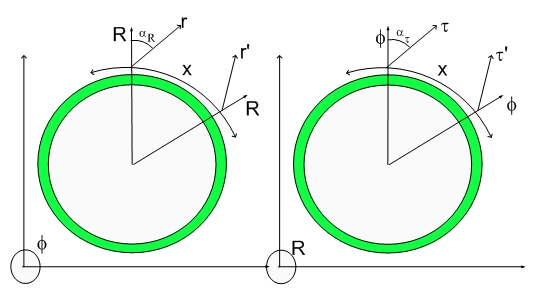Electron-Proton Fundamental Dilator

We will start reviewing the fundamental dilator. This dilator generates the highest Fourier component of the Universe dilaton field. This will become clear from the Hyperon's modeling, but it shouldn't come up as a surprise, since most of the Universe is composed of Protons, Electrons and Protons/Electrons Together (Neutrons).

Below is a figure displaying the Fundamental Dilator or coherence. Notice that I represented a Double Well Potential. This double well potential is created by initial conditions of this 4D deformation. As the Fundamental Universal Fluctuation decayed, it created spinning deformations, being the Electron-Proton dilator a coherence between the two ground states of each potential well. One can think about the Proton-Electron dilator as a slink traveling on a sloped plain. Each side (electron or proton) has a different footprint (4D displacement profile).

Consider that the slink is also rotation (spinning) as it travels downhill and that the 3D space footprint corresponds to the projection along a given direction parallel to the slope gradient. Under these conditions, if the spinning maintains a 2:1 relationship with the slink "walking" step, then the figures below will be easy to understand..:)

Now seriously...:), the above analogy is perfect and easy to imagine, but the drawings below are also equally simple...:)

As in any other propagating disturbance, energy has to be stored in a different state every half cycle. One cannot have a sound wave that is always potential energy (pressure) as doesn't convert into kinetic energy on the next half-cycle. Similarly, the dilaton propagation requires two states where the impacted energy can be stored. One state looks quite like a Proton (3D projection can be proportional to a proton mass), while the other state looks quite like an Electron (3D projection can be proportional to a electron mass). This means that the Electron has a small butt, while the Proton has a big butt. By now, you should be remembering what I said about the Fat Electron.

This is the deformation levels associated with the electron: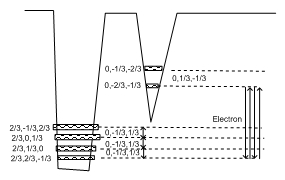Here are the corresponding transitions for the proton: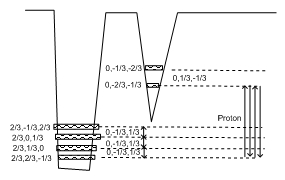Notice that the only difference is the tunneling phase. The electron starts as an electron and the proton starts as a proton!!!!

Notice that in the traditional standard model the electron has no Quarks. The quantum numbers, associated with the 4D deformational states, are modeled as axes’ lengths of a 4D ellipsoid of revolution. Negative values correspond to 180 degrees out of phase with respect to a dilator with a positive axis. This means that when the positive dilator is expanding the 4D space, the negative dilator is shrinking 4D space.

Also present in the diagram are the "excited" deformational states and the spontaneous dynamic symmetry breakdown. States (0,-2/3,-1/3) and (0,-1/3,-2/3) have different "energy" levels and thus slightly different 3D displacement volume projections (different masses).

I mentioned dynamic because, the breakdown should be due to dynamic effects as oppose to other standard symmetry breakdown processes.

The difference is mass is exactly the half-neutrino chord. This will become clearer when the model for the neutron is presented.

Thus the electron is shrinking 4D space while a proton is dilating 4D space. The 3D projection of this 4D shrinking/dilation is equal to the ratio between the two masses (MassOfTheProton/MassOfTheElectron)...:)

The figure also shows that the spinning is half the frequency of the dilation (tunneling between the two wells). This means that while the dilation runs half-cycle, the spinning has only done a quarter-cycle (90 degrees) rotation.

This also means that if a particle starts in phase as a proton, when it becomes an electron it will be sidewise and have zero (very small) 3D projection. This is why for us, an electron is always an electron and a proton is always a proton. The same is not in the 4D space.

I will not explain again the pseudo-quantization of time here because I have to get to the Hyperons, but you have to understand pseudo-quantization of time to understand the Hypergeometrical Standard Model.

Below is another simpler representation of the dilators. Remember that sidewise states cannot be seen in the 3D Universe and that upside states are anti-particles.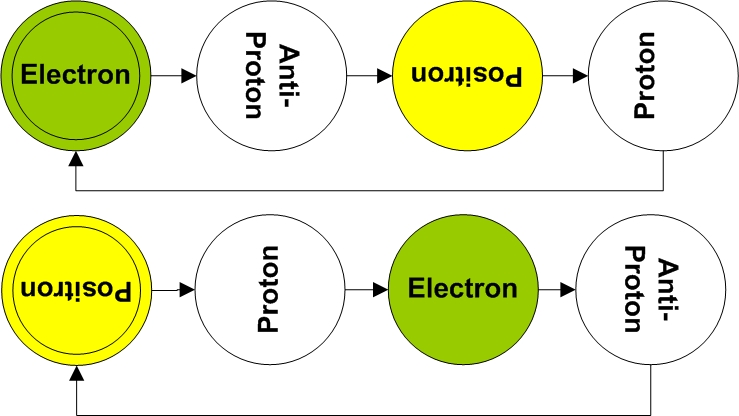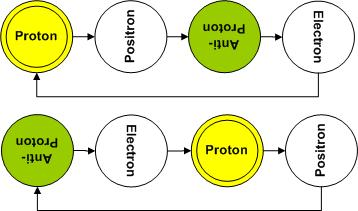The last element of the coherence corresponds to the first state of the next chord. These are like musical chords. The only difference is that smooth space is being deformed instead of air...:)

Thus this chord (proton, electron sidewise, proton upside-down, electron-sidewise) would repeat itself for the life of the particle. In this case, basically forever or until the coherence dephases.

Notice that anti-particle are the same coherences, with an extra rotational phase (180 degrees rotation) with respect to their corresponding particles.

The Neutron

The Neutron Model is the first interesting case and contains the first description of how nuclear energy is stored in deformations of the Fabric of Space.

I will briefly describe it here but I will come back later when I describe how to create coherent nuclear fusion.Notice that the Neutron is composed of a electron and a proton chords. There are in between those two chords, two transmutation notes...:)

The transmutation notes are transitions which add additional dilation phase to the process, thus making the Proton to transmutate from a sidewise proton to an in-phase proton.

Of course, the Neutron model also has a state coherence representation.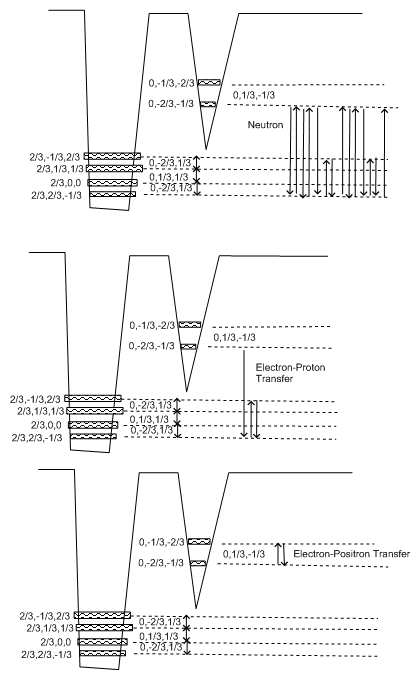Figure above also shows the transitions corresponding to the neutrino and transmutation chords.

These transmutation transitions are related to the neutrinos (of course)...:) and they are approximately half of a neutrino chord each. In the diagram above, you can read the electron chord, followed by a electron-proton transmutation note, followed by a proton chord and finally a proton-electron transmutation note. Notice that the transmutation notes are sub-coherences between proton states and excited electron states. The diagram should indicate clearly the presence of a proton, an electron and an anti-neutrino.... the decay products of a neutron.

Since the phase shift is not perfect, the intra-chord transitions generate a dissonance or a tilted proton/electron or Fabric of Space stress. This stress, which is related to a kinetic state of motion, will result in the phase matching conditions for the decaying products.

This will be reviewed later.

Just for fun, let's see a more complex hyperon...:)

Shown below is a quite complex hyperon: Delta Zero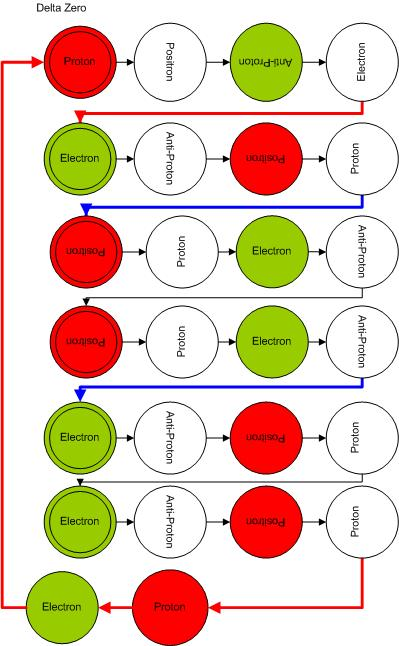It decays onto a pion-zero and a neutron or into a pion minus and a proton...:) and has spin 3/2.

As it should be clear by now, the total charge of this particle is zero. It contains 3 electrons chords, two positrons chords and a proton chord, thus having zero charge. It also contains two electron-proton transmutation notes and two proton-electron transmutation notes. All these transmutation notes are spinning counter-clockwise and they carry a half-spin. One of these chords (sub-coherences) is spinning counter-clockwise while all the others are spinning clockwise. Since each coherence carry half spin, the total spin is 3/2.

I hope you had fun... :)

In the next few blogs, I will start the hyperon family from the bottom up and then you will have the chance to finally understand the whole Universe...:)

MP
Currently unrated

or